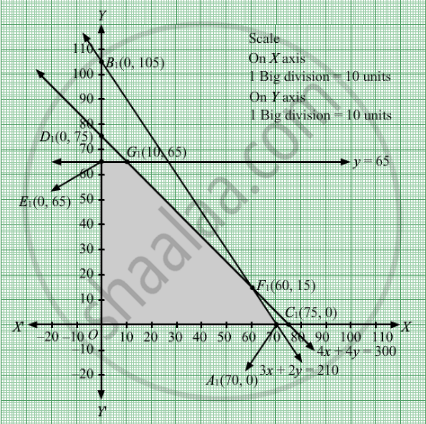# A Firm Manufacturing Two Types of Electric Items, a and B, Can Make a Profit of Rs 20 per Unit of a and Rs 30 per Unit of B. - Mathematics

Sum

A firm manufacturing two types of electric items, A and B, can make a profit of Rs 20 per unit of A and Rs 30 per unit of B. Each unit of A requires 3 motors and 4 transformers and each unit of B requires 2 motors and 4 transformers. The total supply of these per month is restricted to 210 motors and 300 transformers. Type B is an export model requiring a voltage stabilizer which has a supply restricted to 65 units per month. Formulate the linear programing problem for maximum profit and solve it graphically.

#### Solution

Let units of item A and y units of item B were manufactured.
Number of items cannot be negative.
Therefore, $x, y \geq 0$

The given information can be tabulated as follows:

 Product Motors Transformers A(x) 3 4 B(y) 2 4 Availability 210 300

Further, it is given that type B is an export model, whose supply is restricted to 65 units per month.
Therefore, the constraints are

$3x + 2y \leq 210$
$4x + 4y \leq 300$
$y \leq 65$

A and B can make a profit of Rs 20 per unit of A and Rs 30 per unit of B.Therefore, profit gained from x units of item A and y units of item B is Rs 20x and Rs 30y respectively.
Total profit = Z = $20x + 30y$ which is to be maximised.
Thus, the mathematical formulat​ion of the given linear programmimg problem is
Max Z =  $20x + 30y$

subject to

$3x + 2y \leq 210$
$4x + 4y \leq 300$
$y \leq 65$

$x, y \geq 0$

First we will convert inequations into equations as follows:
3x + 2y = 210, 4x + 4y = 300, y = 65, x = 0 and y = 0

Region represented by 3x + 2y ≤ 210:
The line 3x + 2y = 210 meets the coordinate axes at A1(70, 0) and B1(0, 105) respectively. By joining these points we obtain the line 3x + 2y = 210.  Clearly (0,0) satisfies the 3x + 2y = 210. So,the region which contains the origin represents the solution set of the inequation 3x + 2y ≤ 210.

Region represented by 4x + 4y ≤ 300:
The line 4x + 4y = 300 meets the coordinate axes at C1(75, 0) and D1(0, 75) respectively. By joining these points we obtain the line
4x + 4y = 300.Clearly (0,0) satisfies the inequation 4x + 4y ≤ 300. So,the region which contains the origin represents the solution set of the inequation
4x + 4y ≤ 300.

y = 65 is the line passing through the point E1(0, 65) and is parallel to X axis.

Region represented by x ≥ 0 and y ≥ 0:
Since, every point in the first quadrant satisfies these inequations. So, the first quadrant is the region represented by the inequations x ≥ 0, and ≥ 0.
The feasible region determined by the system of constraints 3x + 2y ≤ 210, 4x + 4y ≤ 300, y ≤ 65, x ≥ 0, and y ≥ 0 are as followsThe corner points are O(0, 0),  E1(0, 65), G1

$\left( 10, 65 \right)$, F1(60, 15) and A1(70, 0).

The values of Z at these corner points are as follows

 Corner point Z= 20x + 30y O 0 E1 1950 G1 2150 F1 1650 A1 1400

The maximum value of Z is 2150 which is attained at G1
$\left( 10, 65 \right)$ Thus, the maximum profit is Rs 2150 obtained when 10 units of item A and 65 units of item B were manufactured.
Concept: Graphical Method of Solving Linear Programming Problems
Is there an error in this question or solution?

#### APPEARS IN

RD Sharma Class 12 Maths
Chapter 30 Linear programming
Exercise 30.4 | Q 13 | Page 52